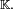# Algebraic geometry

Classical algebraic geometry is the study of geometric properties of the objects defined by algebraic equations. For example, a parabola, such as all solutions (x,y) of the equation yx2 = 0, is one such object, whereas one can prove that the graph of the exponential function—all solutions (x,y) of the equation yex = 0—is not, i.e. the exponential equation cannot be replaced by an equivalent system of polynomial equations. The key distinction is that the equation defining the first example is a polynomial equation, whereas the second cannot be represented by polynomial equations. The first approximation of the adjective algebraic could be defined by polynomials. Next, this kind of algebraic sets are glued together in a proper way to form more general objects of interest to algebraic geometry, while the more elementary algebraic geometry of algebraic sets is closely related to commutative algebra.

## Modern framework

Algebraic geometry developed around classification problems (on the model of the classical theory of conics). But the context of such investigations has undergone considerable evolution since the beginning of the nineteenth century. First the ground field has been extended from the real numbers to the field of complex numbers and later to arbitrary fields. In the second place notions of abstract algebra have led to a natural generalization of algebraic geometry, namely to the concept of algebraic affine variety, a set of points in$\mathbb{K}^n$ that satisfy polynomial equations with coefficients in the field$\mathbb{K}.$

## Reference

J. Dieudonné, A Panoroma of Pure Mathematics, Academic Press, New York, (1982)Some content on this page may previously have appeared on Citizendium.